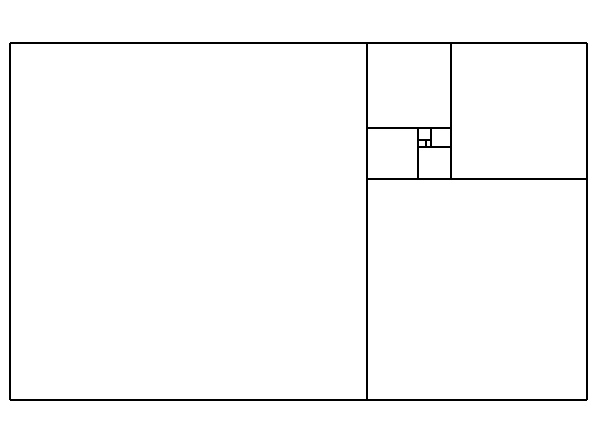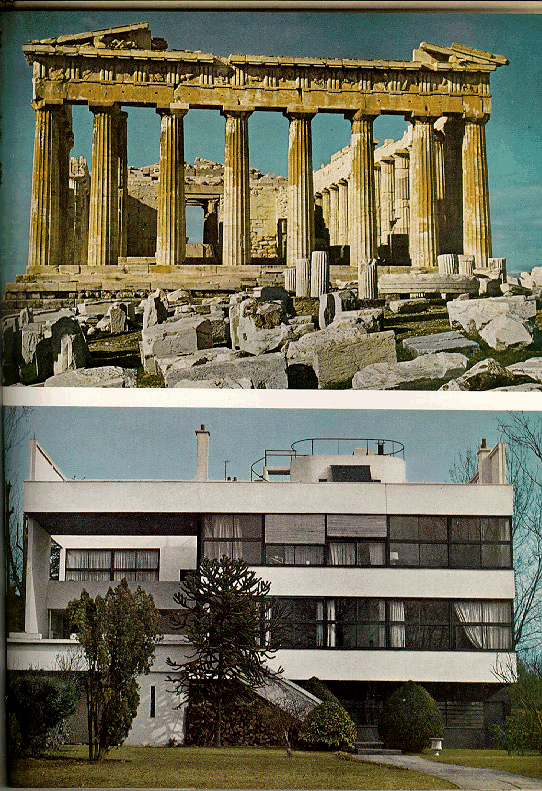### Golden Rectangles

 The golden rectangle R, constructed by the Greeks, has the property that when a square is removed a smaller rectangle of the same shape remains. Thus a smaller square can be removed, and so on, with a spiral pattern resulting.The Greeks were thus able to see geometrically that the sides of R have an irrational ratio, 1 : x. The smaller rectangle has sides with ratio 1-x : 1; since this is the same as the ratio for the big rectangle, one finds that x^2 = x+1 and thus x = (1+Sqrt(5))/2 = 1.618033989.... This golden ratio is also the limit of the ratios of successive Fibonacci numbers, 1,2,3,5,8,13,21,34,55,89,144,..., e.g. 144/89 = 1.61798... The golden rectangle was considered by the Greeks to be of the most pleasing proportions, and its shape figures in ancient architecture.The same motif is used in modern architecture such as the buildings of Le Corbusier (whose only work in North America is the Carpenter Center at Harvard).